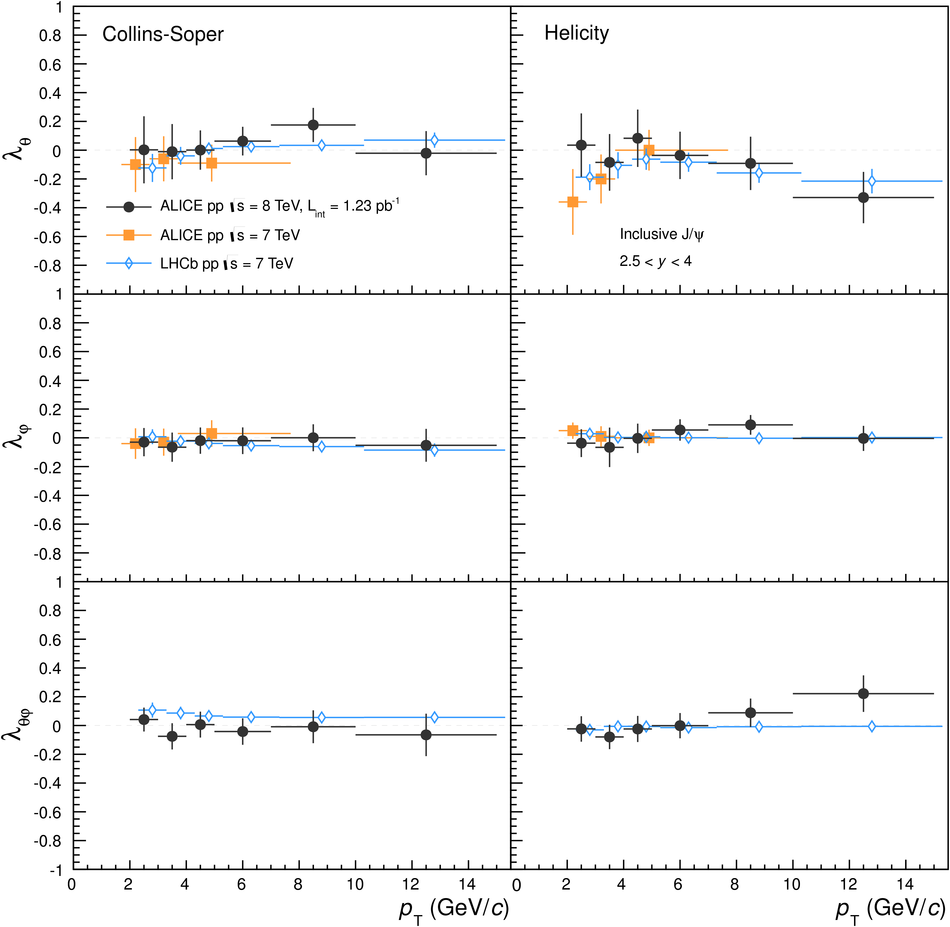# Figure 3

 ALICE inclusive J/$\psi$ polarization parameters in pp collisions at $\sqrt{s} = 8$~TeV (black points) compared with ALICE~ inclusive J/$\psi$ (orange squares, shifted horizontally by $- 0.3$~\GeVc) and LHCb~ prompt J/$\psi$ (blue open diamonds, shifted horizontally by $+ 0.3$~\GeVc) measurements at $\sqrt{s} = 7$~TeV in the rapidity interval $2.5 < y < 4.0$. The error bars represent the total uncertainties. Left and right plots show results in the Collins-Soper and helicity frames, respectively, for $\lambda_\theta$ (top plots), $\lambda_\varphi$ (middle plots) and $\lambda_{\theta\varphi}$ (bottom plots).